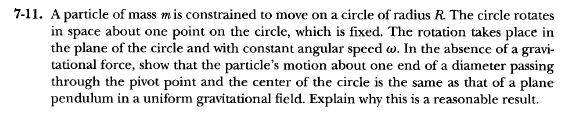# Comparing cicular motion to the motion of a pendulum

Poster has been reminded to use the Template when starting schoolwork threadsThe coordinates of the mass 'm' is $$x=R\cos(\omega{t})+R\cos(\omega{t}+\phi)$$ $$y=R\sin(\omega{t})+R\sin(\omega{t}+\phi)$$
Where $\phi$ is the angle of the particle with respect to coordinate system attached to the circle with origin at center of the circle.

Since particle is not acted upon by any force, So the potential energy can be taken as zero.

The Lagrangian of the system is

$$L=\frac{m}{2}\bigg((R\omega)^2+R^2(\omega+\dot{\phi})^2+2R\omega(\omega+\dot{\phi})\cos{\phi}\bigg)$$

$$J=\frac{\partial L}{\partial \dot{\phi}}=mR^2(\omega+\dot{\phi})+mR^2\omega\cos{\phi}$$

So the equation of motion for $\phi$ coordinate is

$$\ddot{\phi}+{\omega}^2\sin\phi=0$$.

This is the result. I have no doubt regarding this problem. I have doubt regarding total energy of this problem, since the particle is not subjected to any potential, we took $U$=0. So Lagrangian is simply Kinetic energy of the particle, The total energy is the sum of Kinetic energy and potential energy(U=0 for this case), can I infer the Total energy and Lagrangian has the same form?

My actual doubt is Total energy of the particle is not constant of motion, rather $E-\vec{\omega}.\vec{J}$ is the constant of motion. when I derived I got
$$C=E-\vec{\omega}.\vec{J}=\frac{m}{2}\bigg(R^2\dot{\phi}^2+R^2\omega\dot{\phi}\cos{\phi}\bigg)$$

By the definition of Constant of motion, if I take the time derivative of the above quantity, it should give me zero. But I am getting

$$\dot{C}=-mR^2\bigg((\dot{\phi}\omega^2+\omega\dot{\phi}^2)\sin\phi+\omega^3\sin\phi\cos\phi\bigg)\neq{0}$$
I used $$\ddot{\phi}= -{\omega}^2\sin\phi$$ to simplify the above expression.
Is there anywhere I did a mistake, since potential energy is taken as zero, I took total energy as same as Lagrangian, is it wrong? or I messed up somewhere?

#### Attachments

Last edited by a moderator:

BvU
Homework Helper
HI,

Very elegant exercise ! I suspect your mistake is that you assume the forces of constraint do no work. But they do.

•berkeman
TSny
Homework Helper
Gold Member
I got
$$C=E-\vec{\omega}.\vec{J}=\frac{m}{2}\bigg(R^2\dot{\phi}^2+R^2\omega\dot{\phi}\cos{\phi}\bigg)$$
I don't agree with your result for ##C##. I had to be very careful with factors of 2 in the calculation. I do find ##\dot C = 0##

As an intermediate check, what expression did you get for ##\vec{\omega} \cdot \vec J##?

EDIT: I believe that our difference in the result for ##C## is due to a difference in what we used for ##J##. I took ## J## to be the angular momentum of the particle with respect to the axis of rotation of the circular loop as measured in the inertial frame. This is different from your canonical angular momentum ##J = \partial L / \partial \dot {\phi}##.

Last edited:
I don't agree with your result for ##C##. I had to be very careful with factors of 2 in the calculation. I do find ##\dot C = 0##

As an intermediate check, what expression did you get for ##\vec{\omega} \cdot \vec J##?

EDIT: I believe that our difference in the result for ##C## is due to a difference in what we used for ##J##. I took ## J## to be the angular momentum of the particle with respect to the axis of rotation of the circular loop as measured in the inertial frame. This is different from your canonical angular momentum ##J = \partial L / \partial \dot {\phi}##.
Yes I made a small mistake, there is factor of 2 in the second term

$$\vec{\omega}.\vec{J}=mR^2\omega(\omega+\dot{\phi})+mR^2\omega^2\cos(\phi)$$

okay now I understood, I took canonical conguate momentum as my angular momentum with respect to axis of rotation, so J has a different expression? But I have no idea how to find that for this problem, can you give me some hint?

Last edited:
and also wondering what is the significance of the term $$E-\vec{\omega}.\vec{J}$$
why E and J also are individually not constants of motion here?

TSny
Homework Helper
Gold Member
I took canonical conguate momentum as my angular momentum with respect to axis of rotation, so J has a different expression?
Yes.
But I have no idea how to find that for this problem, can you give me some hint?
I used ##\vec J = m \vec r \times \vec v##. You can get ##\vec v## from your expressions for x and y as functions of time.

Last edited:
•Muthumanimaran
TSny
Homework Helper
Gold Member
and also wondering what is the significance of the term $$E-\vec{\omega}.\vec{J}$$
why E and J also are individually not constants of motion here?
The constancy of ##E - \vec \omega \cdot \vec J## was new to me, too. But the following might be a way to see it.

There must be an external torque ##\vec \tau## applied to the system about the axis of rotation of the ring in order to keep the ring rotating at a constant speed. The force that the particle exerts on the ring generally produces a torque on the ring about the axis of rotation. There must be an externally applied torque on the ring in order to keep the net torque on the ring zero so that the angular speed of the ring remains constant. The force that the ring exerts on the particle will cause the angular momentum of the particle (about the axis of rotation) to change. So, we do not expect ##\vec J## to remain constant.

If a force acts on a particle, the rate at which energy is added to the particle by the force is ##\vec F \cdot \vec v##.
Analogously, if a torque acts on a system, the rate at which energy is added to the system by the torque is ##\vec \tau \cdot \vec \omega##.

So, for your system, ##\frac{dE}{dt} = \vec \tau \cdot \vec \omega##. But the applied torque equals the rate of change of angular momentum of the system which equals the rate of change of angular momentum of the particle. So, ##\frac{dE}{dt} = \frac{d \vec J}{dt} \cdot \vec \omega##.

Since ##\vec \omega## is constant, we have ##\frac{dE}{dt} = \frac{d }{dt}\left( \vec J \cdot \vec \omega \right) ## from which we see that ##E - \vec J \cdot \vec \omega## is independent of time.

•Muthumanimaran